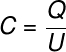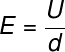Electricity & Megnetism

# Capacitors

Capacitors are electronic devices used for the storage of electrical charges , parallel plate capacitors being the simplest type. In this type of capacitor, dielectric materials are used, linked to different electrical potentials, capable of increasing the amount of charges stored between the capacitor’s armatures.

## What are capacitors?

Capacitors, also known as accumulators, have as their main function the accumulation of electrical charges . They can be found in almost all electrical circuits and are quite simple devices that consist of a dielectric medium that separates two conductive plates connected at different electrical potentials .

## capacitance

Capacitance is a scalar physical quantity that measures the amount of charge that can be stored in a capacitor for a given electrical potential difference. The more charges a capacitor can store, the greater its capacitance .

The unit of measurement for capacitance is the farad (F), or, coulomb per volt (C/V), in the International System of Units ( SI ) units.C – capacitance (F)

Q – electrical charge (C)

U – electric potential (V)

Capacitance is also determined by the shape of the capacitor , as factors such as the area of ​​the capacitor plates and the distance between them determine the magnitude of this quantity. Note how the formula used to calculate the capacitance of a parallel plate capacitor in vacuum looks like:ε 0 – electrical permittivity of vacuum (8.85.10 -12 F/m)

A – area of ​​parallel plates

d – distance between the plates

In the case shown, there is no medium inserted between the capacitor plates. If some dielectric material is inserted between these plates, the capacitance of this device will change, since each medium has its own dielectric characteristic , known as dielectric strength.

## Dielectric strength

Dielectric strength is a property of materials that measures the voltage limit to which a given dielectric medium needs to be subjected to become a conductive medium . In the following table, we show the dielectric strength values ​​for some known material media.

 Material Dielectric strength (V/m) Air 3.10 6 Paper 16.10 6 Teflon 60.10 6 Eraser 12.10 6 Porcelain 12.10 6 Wood ~10.10 6 Oil (transformer) 20 – 30.10 6

## Electric field

It is possible to calculate the electric field between the plates of the capacitor, for that, we only need to know the distance between the plates and the potential difference between them. Watch:E – electric field (V/m or N/C)

## polarization of charges

When a large electric field is established through dielectric materials, they polarize, that is, they start to show a small separation of charges in their molecules . Charges with a negative sign move towards the positive plate of the capacitor and this gives rise to a polarizing electric field inside the dielectric, contrary to the external electric field .

The operation is basically as follows: the greater the dielectric strength between the plates, the greater the amount of electrical charges that can be polarized, thus, the more charges can be stored between the capacitor’s armatures.